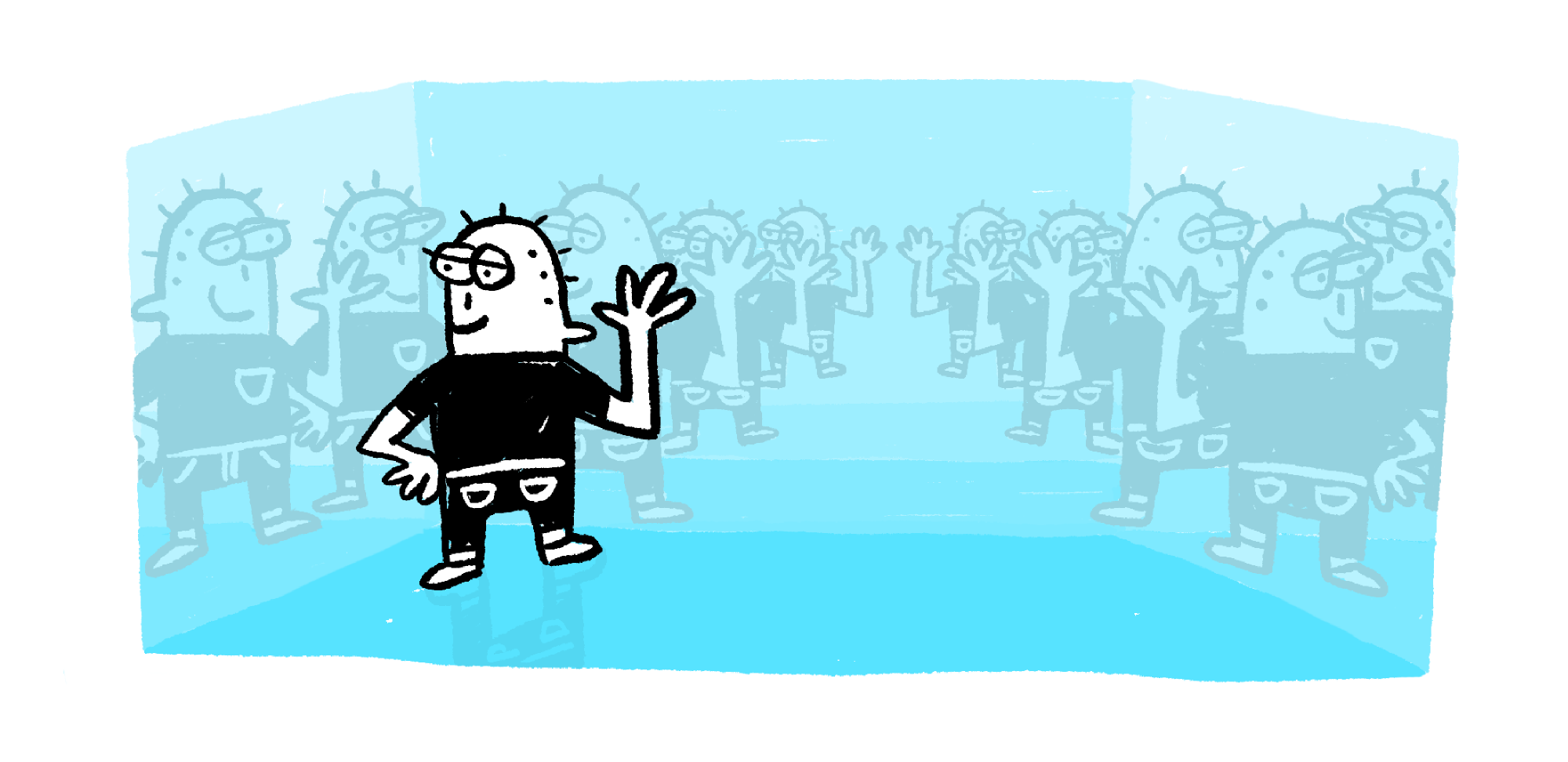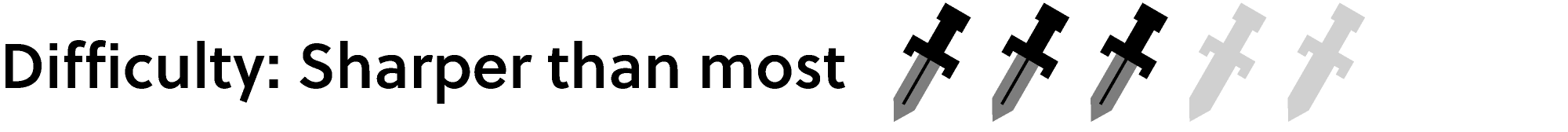Back

## House of Mirrors

Covering the walls of a room with mirrors exposes an infinite abyss — an explosion of reflections trailing off as far as the eye can see!Between bounces, light usually travels in straight lines called rays. This fact is hardwired into your brain, and it's how you form a mental picture of the world around you from an image.

What exactly happens when a ray of light hits a mirror? Smooth surfaces, like polished glass or metal, reflect almost all light that lands on them.

A light ray that hits a mirror is traveling in one direction before the reflection and in a different direction afterwards. These directions obey a simple relationship.

Let's follow a ray of light coming toward a mirror at an angle. To figure out which direction it goes after it's reflected, we first draw a line that makes a right angle to the mirror's surface (the dashed line on the diagram below).

The angle between the incoming ray and the dashed line is called the angle of incidence $\theta_\text{i}.$ The angle between the ray after it's reflected is $\theta_\text{r}.$ The reflected ray always makes the same angle with the dashed line as the incoming ray: $\theta_\text{i}=\theta_\text{r}$. This is often stated as the law of reflection:

Law of reflection: The angle of incidence is equal to the angle of reflection.

For a ray that's reflected just once, this law seems pretty uninteresting. But in a house of mirrors or a kaleidoscope, a ray may bounce hundreds of times before you see it. Even so, this law is so accurate that you can pinpoint its exact source.

For example, here is a box with three mirrored walls. The fourth wall (on the right) is covered by three light sources — LED panels that emit red, green, and blue lights.

A thin eyepiece, which makes an angle of $60^\circ$ with the bottom wall, lets you view any ray parallel to the long axis of the eyepiece. What color do you see?

The source of the ray entering the eyepiece can be found by tracing it backwards using the law of reflection. You can adjust the sliders on the diagram to ensure that the law is satisfied for each reflection from the source to the eyepiece.

Since the eyepiece makes a $60^\circ$ with the bottom wall, the angle of reflection of the ray that bounces off the top wall is $90^\circ-60^\circ=30^\circ.$ The incident angle equals the reflected angle, so the incident angle of the first bounce $\theta_1$ is $30^\circ$ (which we've set for you already).

If you continue tracing the ray backwards, setting the reflected angle equal to the incident angle for each reflection, you'll find that the source of the ray entering the eyepiece is the $\textcolor{#20A900}{\text{green}}$ light!

Reflection isn't the only behavior of light when it encounters an object — it can also be absorbed. Now that you have the mechanics of adjusting the reflected rays on the diagram, try to solve Today's Challenge featuring a box both reflecting and absorbing surfaces.

# Today's Challenge

Sources of red, green, and blue lights cover one of the walls of this box. Five other walls are mirrored. The last two walls are painted black and absorb all light. If a ray touches an absorbing wall, it ends.

The eyepiece in this box can be set to $6$ different angles. Trace the ray that enters the eyepiece at each setting to its source. The black line is a tool to help you trace the path.

Which colors can you see through the eyepiece? (You may select more than one color.)Select one or more

×